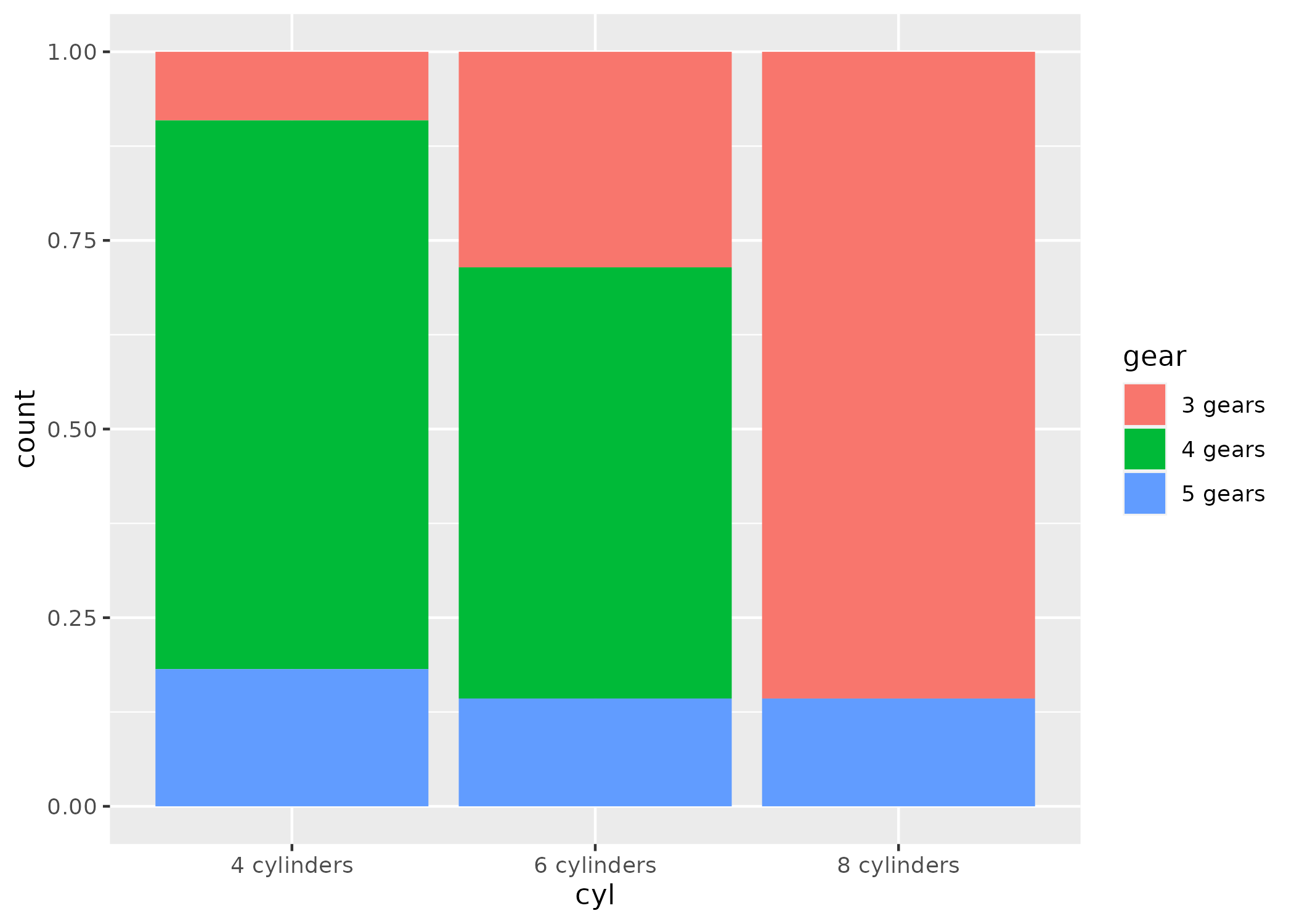# Plotting amounts: barcharts and dot plots

Author

Jeffrey R. Stevens

Published

April 14, 2023

1. Using the `mtcars` data, create a barchart of the counts for each level of cylinder.
``# >``
1. Repeat the barchart but stack the counts by gear.
``# >``
1. Recreate this plot:``# >``
1. Repeat barchart #2 but set the position to “dodge”.
``# >``
1. Whoa, what happened to 8 cylinders? Unfortunately, since there were only two levels of gear for 8 cylinders, it just split the bars in two. To hold the numbers of bars the same across all levels, you can set position to `position_dodge(preserve = "single")`. Try that.
``# >``
1. Well, that’s better—the two bars are the same width as all of the other bars. But the 4 gears should show up as 0. To fix, we need to count the data first, find implicitly missing data, and plot using `geom_col()`. So first, find counts for the combinations of cylinders and gears. Then use `complete()` to find the implicitly missing combinations. Then replace the NAs with 0s. Then use `geom_col()` to plot these values with the position dodged.
``# >``
1. Make a dotplot of the counts for each level of carb and plot carb on the y-axis and the count on the x-axis. Reminder that first you’ll need to count the observations in each level of carb before starting the plot.
``# >``
1. Repeat dotplot #8 but order carb based on the counts from highest to lowest count.
``# >``